Next: Worked example 4.1: In Up: Newton's laws of motion Previous: Friction

## Frames of reference

As discussed in Sect. 1, the laws of physics are assumed to possess objective reality. In other words, it is assumed that two independent observers, studying the same physical phenomenon, would eventually formulate identical laws of physics in order to account for their observations. Now, two completely independent observers are likely to choose different systems of units with which to quantify physical measurements. However, as we have seen in Sect. 1, the dimensional consistency of valid laws of physics renders them invariant under transformation from one system of units to another. Independent observers are also likely to choose different coordinate systems. For instance, the origins of their separate coordinate systems might differ, as well as the orientation of the various coordinate axes. Are the laws of physics also invariant under transformation between coordinate systems possessing different origins, or a different orientation of the various coordinate axes?

Consider the vector equation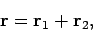(116)

which is represented diagrammatically in Fig. 12. Suppose that we shift the origin of our coordinate system, or rotate the coordinate axes. Clearly, in general, the components of vectors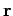,, andare going to be modified by this change in our coordinate scheme. However, Fig. 12 still remains valid. Hence, we conclude that the vector equation (116) also remains valid. In other words, although the individual components of vectors,, andare modified by the change in coordinate scheme, the interrelation between these components expressed in Eq. (116) remains invariant. This observation suggests that the independence of the laws of physics from the arbitrary choice of the location of the underlying coordinate system's origin, or the equally arbitrary choice of the orientation of the various coordinate axes, can be made manifest by simply writing these laws as interrelations between vectors. In particular, Newton's second law of motion,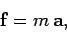(117)

is clearly invariant under shifts in the origin of our coordinate system, or changes in the orientation of the various coordinate axes. Note that the quantity(i.e., the mass of the body whose motion is under investigation), appearing in the above equation, is invariant under any changes in the coordinate system, since measurements of mass are completely independent of measurements of distance. We refer to such a quantity as a scalar (this is an improved definition). We conclude that valid laws of physics must consist of combinations of scalars and vectors, otherwise they would retain an unphysical dependence on the details of the chosen coordinate system.

Up to now, we have implicitly assumed that all of our observers are stationary (i.e., they are all standing still on the surface of the Earth). Let us, now, relax this assumption. Consider two observers,and, whose coordinate systems coincide momentarily at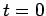. Suppose that observeris stationary (on the surface of the Earth), whereas observermoves (with respect to observer) with uniform velocity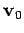. As illustrated in Fig. 34, ifrepresents the displacement of some body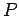in the stationary observer's frame of reference, at time, then the corresponding displacement in the moving observer's frame of reference is simply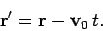(118)

The velocity of bodyin the stationary observer's frame of reference is defined as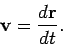(119)

Hence, the corresponding velocity in the moving observer's frame of reference takes the form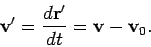(120)

Finally, the acceleration of bodyin stationary observer's frame of reference is defined as(121)

whereas the corresponding acceleration in the moving observer's frame of reference takes the form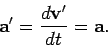(122)

Hence, the acceleration of bodyis identical in both frames of reference.It is clear that if observerconcludes that bodyis moving with constant velocity, and, therefore, subject to zero net force, then observerwill agree with this conclusion. Furthermore, if observerconcludes that bodyis accelerating, and, therefore, subject to a force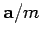, then observerwill remain in agreement. It follows that Newton's laws of motion are equally valid in the frames of reference of the moving and the stationary observer. Such frames are termed inertial frames of reference. There are infinitely many inertial frames of reference--within which Newton's laws of motion are equally valid--all moving with constant velocity with respect to one another. Consequently, there is no universal standard of rest in physics. Observermight claim to be at rest compared to observer, and vice versa: however, both points of view are equally valid. Moreover, there is absolutely no physical experiment which observercould perform in order to demonstrate that he/she is at rest whilst observeris moving. This, in essence, is the principle of special relativity, first formulated by Albert Einstein in 1905.Next: Worked example 4.1: In Up: Newton's laws of motion Previous: Friction
Richard Fitzpatrick 2006-02-02